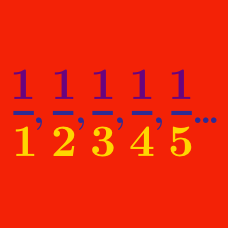Calculus

# Limits of Sequences: Level 2 Challenges

Tetration is defined as

$\Large {^{n}a} = \underbrace{a^{a^{\cdot^{\cdot^{a}}}}}_{n \ a\text{'s}}.$

Find the value of

$\lim_{n\rightarrow\infty}{^{n}}\big(\sqrt {2}\big).$

Consider the sequence $a_1,a_2,\ldots$ where $a_n = \cos(π\sqrt{n^{2}+n})$. Find $\large \lim_{n\rightarrow\infty} a_n.$

Consider the sequence $\left\{a_n\right\}$ where $a_n=\left(\frac{2x-1}{5}\right)^n.$ What is the sum of all integers $x$ for which this sequence converges?

Let $P_{n}$ be the product of the numbers in the $n$th row of Pascal's Triangle.
$\lim_{n\to\infty}\frac{P_{n-1} P_{n+1}}{P_{n}^2} =$

The sequence $\{a_n\}$ follows the recursion $a^2_{n+1}=2a_n+3$ with $a_1=7.$

Determine $\displaystyle \lim_{n \to \infty} a_n$.

×